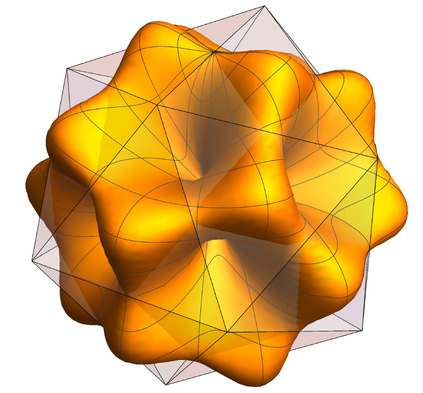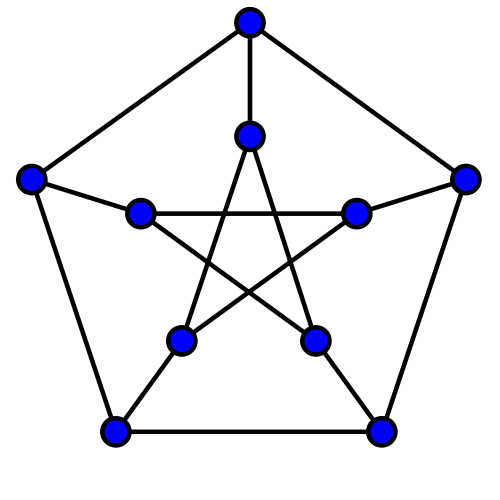# Getting started with Sum of Squares

## Documentation and examples on using Sum of Squares solvers, tutorial and examples of Sum of Squares programmingHere is a list of exercises to be used during the AMS Short Course “Sum of Squares: Theory and Applications”.

## Exercise 1

Consider the polynomial $$p(x) = x^4 + 2 a x^2 + b$$. For what values of $$(a,b)$$ is this polynomial nonnegative? Draw the region of nonnegativity in the $$(a,b)$$ plane. Where does the discriminant of $$p$$ vanish? How do you explain this?

## Exercise 2

Let $$M(x,y,z) = x^4 y^ 2+ x^2 y^4 + z^6 - 3 x^2 y^2 z^2$$ be the Motzkin polynomial. Show that $$M(x,y,z)$$ is not SOS, but $$(x^2+y^2+z^2) \cdot M(x,y,z)$$ is.

## Exercise 3

Give a rational certificate of the nonnegativity of the trigonometric polynomial $$p(\theta) = 5 - \sin \theta + \sin 2\theta - 3 \cos 3\theta$$.

## Exercise 4

Consider a univariate polynomial of degree $$d$$, that is bounded by one in absolute value on the interval $$[-1,1]$$. How large can its leading coefficient be? Give an SOS formulation for this problem, solve it numerically for $$d=2,3,4,5$$, and plot the found solutions. Can you guess what the general solution is as a function of $$d$$? Can you characterize the optimal polynomial?

## Exercise 5

Consider a given univariate rational function $$r(x)$$, for which we want to find a good polynomial approximation $$p(x)$$ of fixed degree $$d$$ on the interval $$[-2,2]$$.

1. Write an SOS formulation to compute the best polynomial approximation of $$r(x)$$ in the supremum norm.
2. Same as before, but now $$p(x)$$ is also required to be convex.
3. Same as before, but $$p(x)$$ is required to be a convex lower bound of $$r(x)$$ (i.e., $$p(x) \leq r(x)$$ for all $$x \in [-2,2]$$).
4. Let $$r(x) = \frac{1-2 x+x^2}{1+x+x^2}$$. Find the solution of the previous subproblems (for $$d=4$$), and plot them.

## Exercise 6

Consider the polynomial system $$\{ x+y^3 =2, x^2+y^2=1\}$$.

1. Is it feasible over $$\mathbb{C}$$? How many solutions are there?
2. Is it feasible over $$\mathbb{R}$$? If not, give a Positivstellensatz-based infeasibility certificate of this fact.

## Exercise 7

Consider the butterfly curve in $$\mathbb{R}^2$$, defined by the equation

$x^6 + y^6 = x^2.$

Give an sos certificate that the real locus of this curve is contained in a disk of radius $$5/4$$. Is this the best possible constant?

## Exercise 8

Consider the quartic form in four variables

$p(w,x,y,z) := w^4 + x^2y^2 + x^2z^2 + y^2z^2 - 4wxyz.$
1. Show that $$p(w, x, y, z)$$ is not a sum of squares.
2. Find a multiplier $$q(w,x,y,z)$$ such that $$q(w,x,y,z) p(w,x,y,z)$$ is a sum of squares.

# Exercise 9

Consider a random variable $$X$$, with an unknown probability distribution supported on the set $$\Omega$$, and for which we know its first $$d+1$$ moments $$(\mu_0,\ldots,\mu_d)$$. We want to find bounds on the probability of an event $$S \subseteq \Omega$$, i.e., want to bound $$P(X \in S)$$. We assume $$S$$ and $$\Omega$$ are given intervals. Consider the following optimization problem in the decision variables $$c_k$$:

$\text{minimize} \sum_{k=0}^d c_k \mu_k \qquad \mbox{s.t.} \quad \begin{cases} \sum_{k=0}^d c_k x^k \geq 1 & \forall x \in S \\ \sum_{k=0}^d c_k x^k \geq 0 & \forall x \in \Omega. \end{cases}$
1. Show that any feasible solution of this problem gives a valid upper bound on $$P(X \in S)$$. How would you solve this problem?

2. Assume that $$\Omega = [0,5]$$, $$S = [4,5]$$, and we know that the mean and variance of $$X$$ are equal to $$1$$ and $$1/2$$, respectively. Give upper and lower bounds on $$P(X \in S)$$. Are these bounds tight? Can you find the worst-case distributions?

# Exercise 10

The stability number $$\alpha(G)$$ of a graph $$G$$ is the cardinality of its largest stable set. Define the ideal $$I = \langle x_i (1-x_i) \quad i \in V, \quad x_i x_j \quad (i,j) \in E \rangle$$.

1. Show that $$\alpha(G)$$ is exactly given by
$\min \gamma \qquad \gamma - \sum_{i \in V} x_i \quad \text{is SOS mod I}.$

[Hint: recall (or prove!) that if $$I$$ is zero-dimensional and radical, then $$p(x) \geq 0$$ on $$V(I)$$ if and only if $$p(x)$$ is SOS mod $$I$$.]

1. Recall that a polynomial is 1-SOS if it can be written as a sum of squares of affine (degree 1) polynomials. Show that an upper bound on $$\alpha(G)$$ can be obtained by solving
$\min \gamma \qquad \gamma - \sum_{i \in V} x_i \quad \text{is 1-SOS mod I}.$
1. Show that the given generators of the ideal $$I$$ are already a Gröbner basis. Show that there is a natural bijection between standard monomials and stable sets of $$G$$.

2. As a consequence of the previous fact, show that $$\alpha(G)$$ is equal to the degree of the Hilbert function of $$\mathbb{R}[x]/I$$.

Now let $$G =(V,E)$$ be the Petersen graph, shown below.1. Find a stable set in the Petersen graph of maximum cardinality.

2. Solve this optimization problem for the Petersen graph. What is the corresponding upper bound?

3. Compute the Hilbert function of $$I$$ using Macaulay2, and verify that this answer is consistent with your previous results.

# Exercise 11

Consider linear maps between symmetric matrices, i.e., of the form $$\Lambda:\mathcal{S}^n \rightarrow \mathcal{S}^m$$. A map is said to be a positive map if it maps the PSD cone $$S^n_+$$ into the PSD cone $$S^m_+$$ (i.e., it preserves positive semidefinite matrices).

1. Show that any linear map of the form $$A \mapsto \sum_i P_i^T A P_i$$, where $$P_i \in \mathbb{R}^{n \times m}$$, is positive. These maps are known as decomposable maps.

2. Show that the linear map $$C:\mathcal{S}^3 \rightarrow \mathcal{S}^3$$ (due to M.-D. Choi) given by:

$C:A \mapsto \begin{bmatrix} 2 a_{11} + a_{22} & 0 & 0 \\ 0 & 2 a_{22} + a_{33} & 0 \\ 0 & 0 & 2 a_{33} + a_{11} \end{bmatrix} - A.$

is a positive map, but is not decomposable.

Hint: Consider the polynomial defined by $$p(x,y) := y^T \Lambda(x x^T) y$$. How can you express positivity and decomposability of the linear map $$\Lambda$$ in terms of the polynomial $$p$$?

End of exercises.